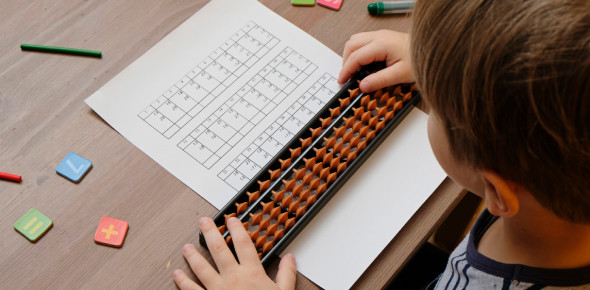# Mental Maths Quiz For Class 5 With Answers

14 Questions | Attempts: 15936SettingsEvery person has a certain mental capability and IQ. This 'mental maths quiz for class 5' has been designed to check how well you are in solving mathematical problems without using any calculator or gadget. So, brush up on your mental math skills by attempting this quiz. There is a list of word problems and calculations. Read the questions carefully and answer. So, let's try out the quiz. Good Luck!

• 1.
What is 2+2?
• A.

3

• B.

4

• C.

65

• D.

11

• 2.
What is 44+44?
• A.

78

• B.

88

• C.

89

• D.

76

• 3.
What is 50x2?
• A.

52

• B.

50x

• C.

(504x4)/45+4

• D.

100

• 4.
What is 33x3?
• A.

36

• B.

99

• C.

100

• D.

98

• 5.
What is 100-50?
• A.

50

• B.

150

• C.

25

• D.

5,000

• 6.
What is 45-32?
• A.

23

• B.

3

• C.

13

• D.

25

• 7.
What is 400/5
• A.

80

• B.

20

• C.

395

• D.

200

• 8.
What is what is 44-23?
• A.

21

• B.

22

• C.

23

• D.

24

• 9.
Use X=6, Y=3 and N=10 for this problem. X+Y=?
• A.

10

• B.

11

• C.

9

• D.

3.14

• 10.
Use X=6, Y=3 and N=10 for this problem. What is NxNxY?
• A.

3

• B.

30

• C.

300

• D.

3,000

• 11.
One the tree there were 10 apples. 3 fell off, Bob picked 4 and Sally picked another, how many were left?
• A.

3

• B.

2

• C.

4

• D.

1

• 12.
In Mr. Roger'class there were 20 students. How many limbs, not counting Mr. Rodger's, were in the room with everyone in class?
• A.

80

• B.

60

• C.

40

• D.

What's a limb?

• 13.
30+70=
• A.

100

• B.

120

• C.

110

• D.

140

• 14.
90+1+9-10+10-10+10-50/2=
• A.

25

• B.

35

• C.

45

• D.

49

## Related TopicsBack to top
×

Wait!
Here's an interesting quiz for you.